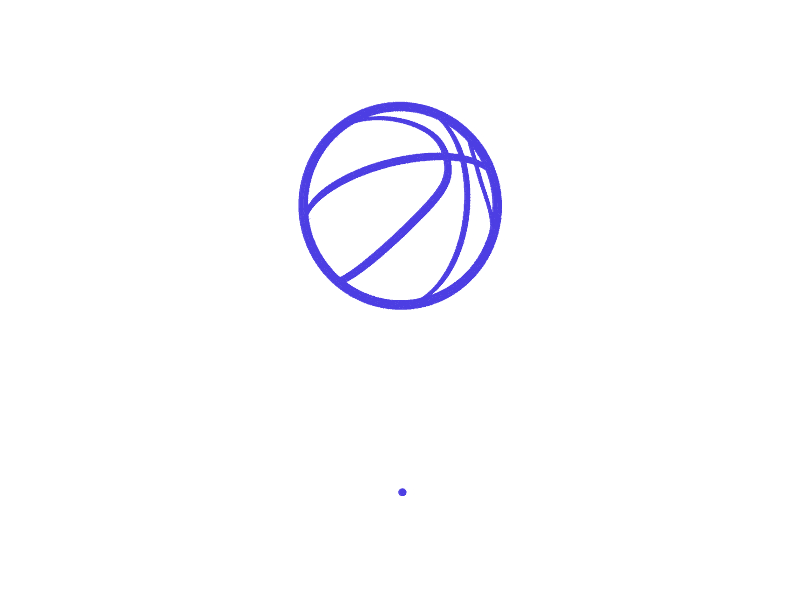# I MAKETHINGS.

Hi, my name is Ishaan Mishra and I'm a multidisciplinary Creative Director and Storyteller based in New York City. I have over a decade of experience in the design and digital media industry. Currently working as Design Director at TikTok. Read more about me, check out some of my work below and reach out if you are interested in collaborating.

SCROLL DOWN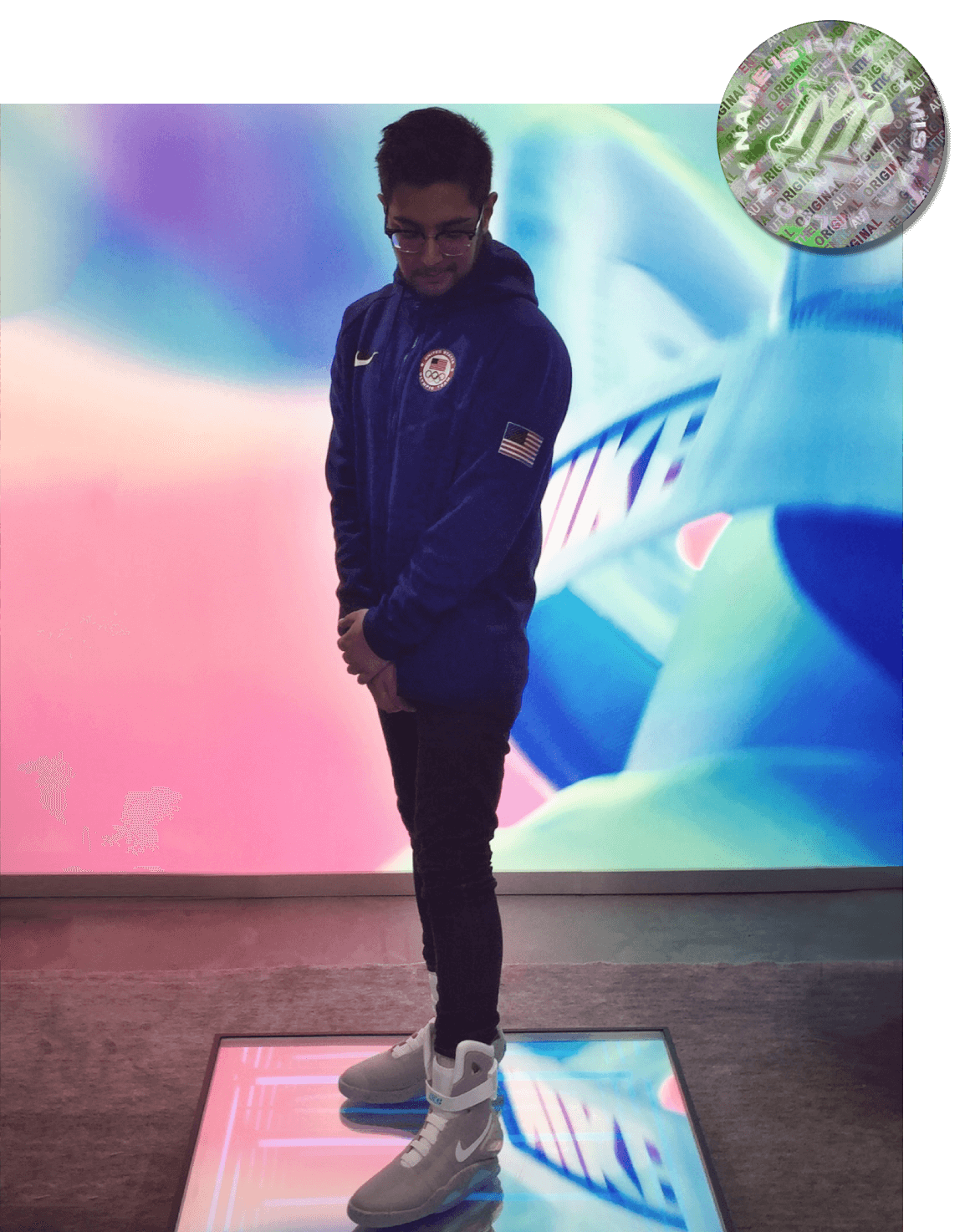☺ LET'S WORK TOGETHER. ☺          ☺ AVAILABLE FOR PROJECTS NOW. ☺          ☺ LET'S WORK TOGETHER. ☺          ☺ AVAILABLE FOR PROJECTS NOW. ☺          ☺ LET'S WORK TOGETHER. ☺          ☺ AVAILABLE FOR PROJECTS NOW. ☺          ☺ LET'S WORK TOGETHER. ☺          ☺ AVAILABLE FOR PROJECTS NOW. ☺          ☺ LET'S WORK TOGETHER. ☺          ☺ AVAILABLE FOR PROJECTS NOW. ☺          ☺ LET'S WORK TOGETHER. ☺          ☺ AVAILABLE FOR PROJECTS NOW. ☺          ☺ LET'S WORK TOGETHER. ☺          ☺ AVAILABLE FOR PROJECTS NOW. ☺          ☺ LET'S WORK TOGETHER. ☺          ☺ AVAILABLE FOR PROJECTS NOW. ☺          ☺ LET'S WORK TOGETHER. ☺          ☺ AVAILABLE FOR PROJECTS NOW. ☺          ☺ LET'S WORK TOGETHER. ☺          ☺ AVAILABLE FOR PROJECTS NOW. ☺          ☺ LET'S WORK TOGETHER. ☺          ☺ AVAILABLE FOR PROJECTS NOW. ☺          ☺ LET'S WORK TOGETHER. ☺          ☺ AVAILABLE FOR PROJECTS NOW. ☺          ☺ LET'S WORK TOGETHER. ☺          ☺ AVAILABLE FOR PROJECTS NOW. ☺          ☺ LET'S WORK TOGETHER. ☺          ☺ AVAILABLE FOR PROJECTS NOW. ☺          ☺ LET'S WORK TOGETHER. ☺          ☺ AVAILABLE FOR PROJECTS NOW. ☺          ☺ LET'S WORK TOGETHER. ☺          ☺ AVAILABLE FOR PROJECTS NOW. ☺          ☺ LET'S WORK TOGETHER. ☺          ☺ AVAILABLE FOR PROJECTS NOW. ☺          ☺ LET'S WORK TOGETHER. ☺          ☺ AVAILABLE FOR PROJECTS NOW. ☺          ☺ LET'S WORK TOGETHER. ☺          ☺ AVAILABLE FOR PROJECTS NOW. ☺          ☺ LET'S WORK TOGETHER. ☺          ☺ AVAILABLE FOR PROJECTS NOW. ☺          ☺ LET'S WORK TOGETHER. ☺          ☺ AVAILABLE FOR PROJECTS NOW. ☺          ☺ LET'S WORK TOGETHER. ☺          ☺ AVAILABLE FOR PROJECTS NOW. ☺          ☺ LET'S WORK TOGETHER. ☺          ☺ AVAILABLE FOR PROJECTS NOW. ☺          ☺ LET'S WORK TOGETHER. ☺          ☺ AVAILABLE FOR PROJECTS NOW. ☺          ☺ LET'S WORK TOGETHER. ☺          ☺ AVAILABLE FOR PROJECTS NOW. ☺          ☺ LET'S WORK TOGETHER. ☺          ☺ AVAILABLE FOR PROJECTS NOW. ☺          ☺ LET'S WORK TOGETHER. ☺          ☺ AVAILABLE FOR PROJECTS NOW. ☺          ☺ LET'S WORK TOGETHER. ☺          ☺ AVAILABLE FOR PROJECTS NOW. ☺          ☺ LET'S WORK TOGETHER. ☺          ☺ AVAILABLE FOR PROJECTS NOW. ☺          ☺ LET'S WORK TOGETHER. ☺          ☺ AVAILABLE FOR PROJECTS NOW. ☺          ☺ LET'S WORK TOGETHER. ☺          ☺ AVAILABLE FOR PROJECTS NOW. ☺          ☺ LET'S WORK TOGETHER. ☺          ☺ AVAILABLE FOR PROJECTS NOW. ☺          ☺ LET'S WORK TOGETHER. ☺          ☺ AVAILABLE FOR PROJECTS NOW. ☺          ☺ LET'S WORK TOGETHER. ☺          ☺ AVAILABLE FOR PROJECTS NOW. ☺          ☺ LET'S WORK TOGETHER. ☺          ☺ AVAILABLE FOR PROJECTS NOW. ☺          ☺ LET'S WORK TOGETHER. ☺          ☺ AVAILABLE FOR PROJECTS NOW. ☺          ☺ LET'S WORK TOGETHER. ☺          ☺ AVAILABLE FOR PROJECTS NOW. ☺          ☺ LET'S WORK TOGETHER. ☺          ☺ AVAILABLE FOR PROJECTS NOW. ☺          ☺ LET'S WORK TOGETHER. ☺          ☺ AVAILABLE FOR PROJECTS NOW. ☺          ☺ LET'S WORK TOGETHER. ☺          ☺ AVAILABLE FOR PROJECTS NOW. ☺

## TESTIMONIALS

01

Ishaan is the absolute best innovator I know. He has always pushed the limits when it comes to content and disruption.

Omar Raja
(Founder, House of Highlights)

02

I associate the words creativity and Ishaan hand and hand. He knows how to really bring an artistic vision to life.

Duane Jackson
(Director of Celebrity Relations, FaZe Clan)

03

Ishaan reinvented the look and feel of social highlights, dude is a baller, made Bleacher Report what it is today.

(Host,Inside the NBA on TNT)

04

Ishaan brings the secret sauce that elevates every piece of content he touches with his creative vision and leadership.

(Senior Vice President, SB Nation/Vox)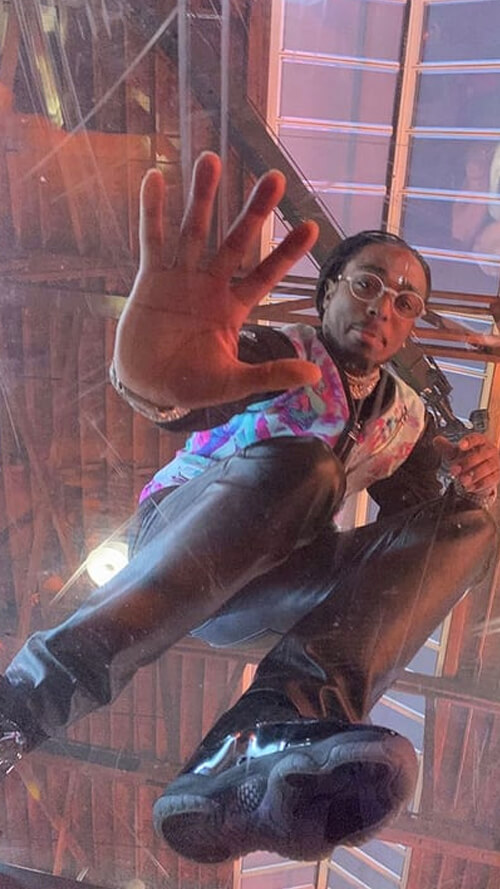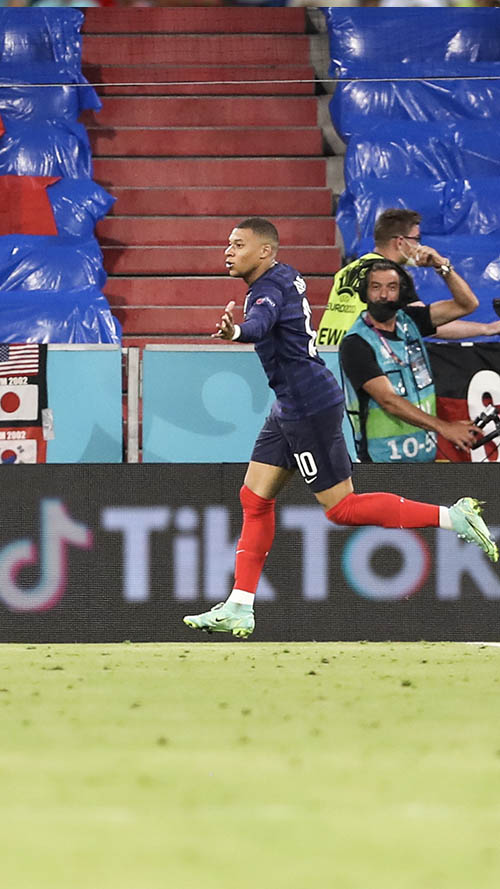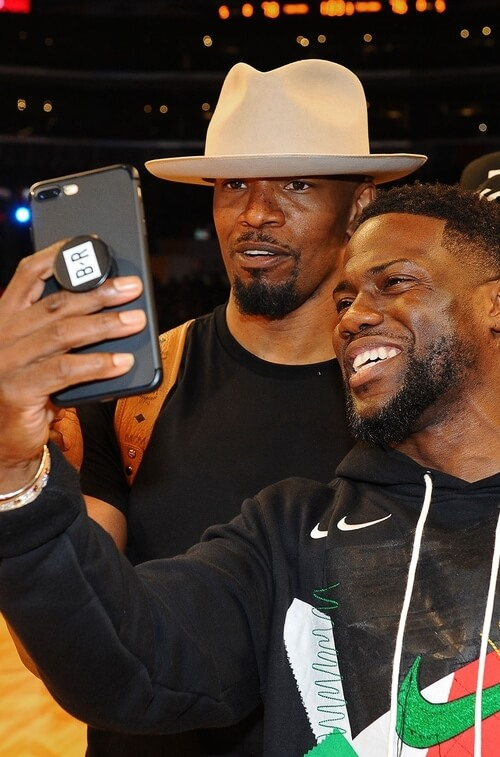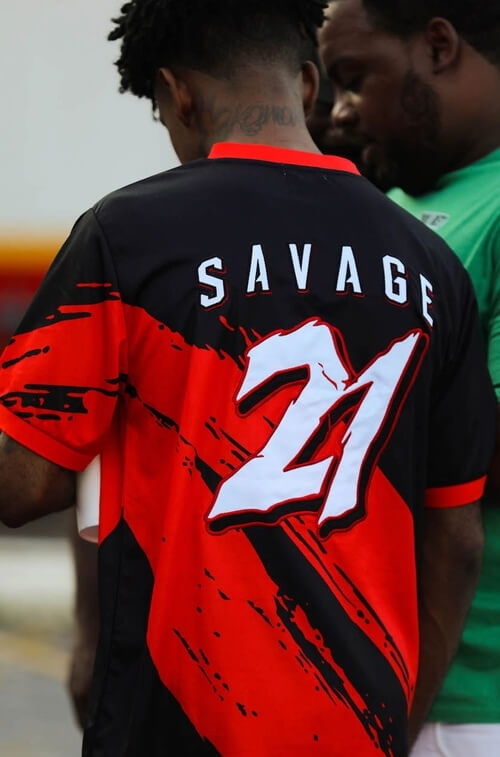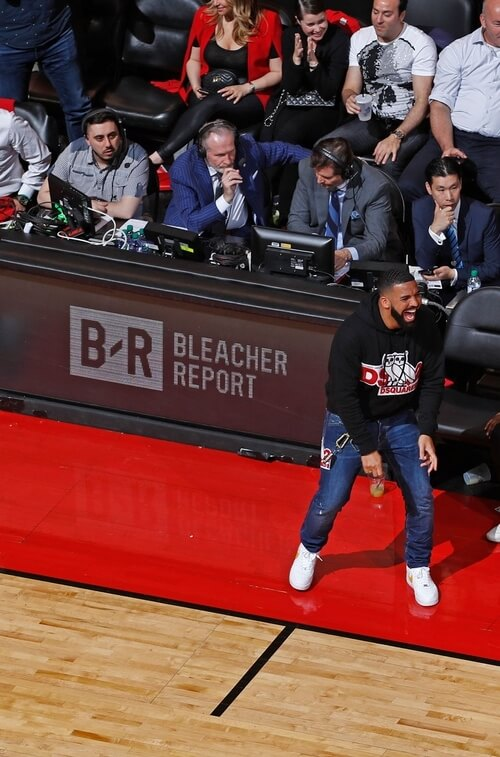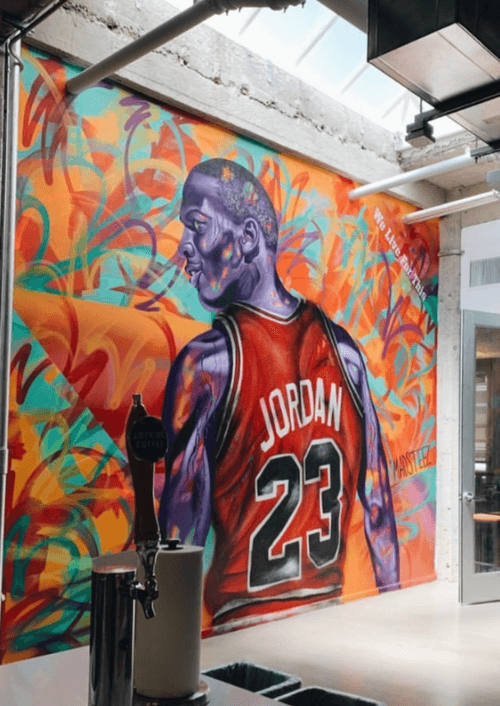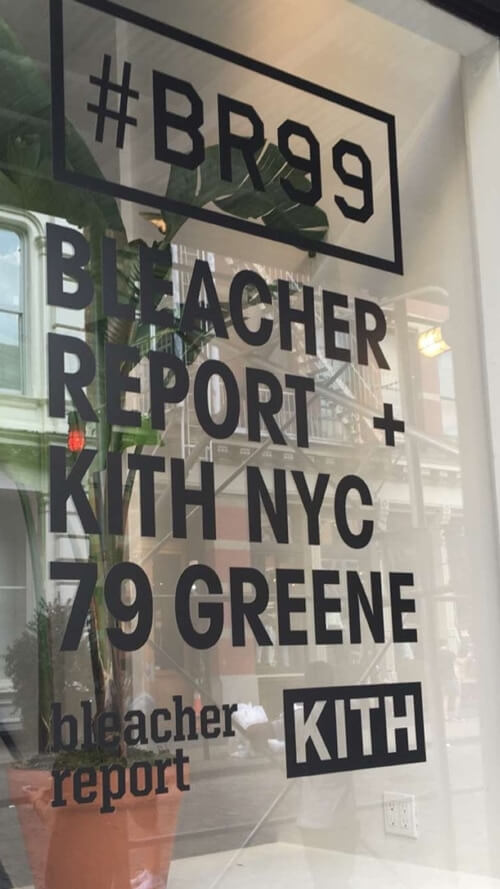294
Projects
12
years
18k+
hours
38
awards

## WORKED WITH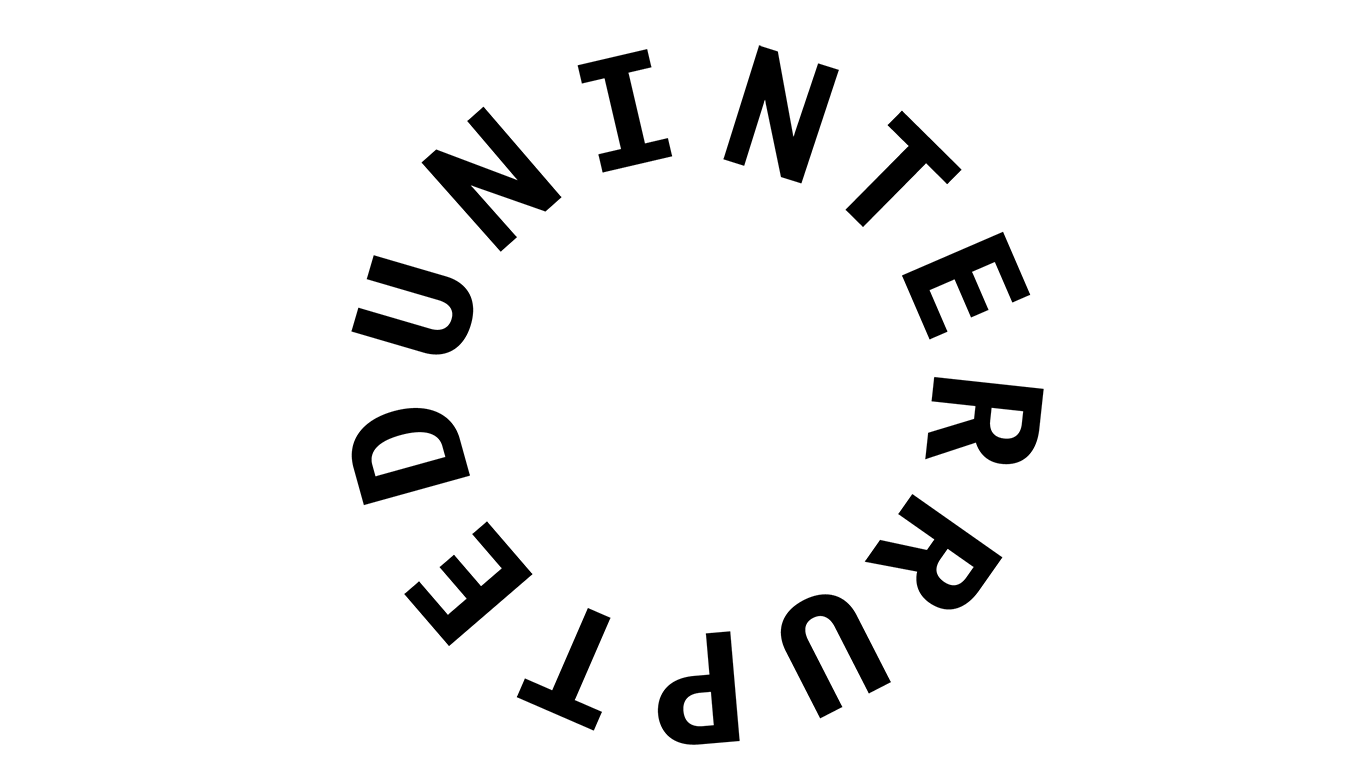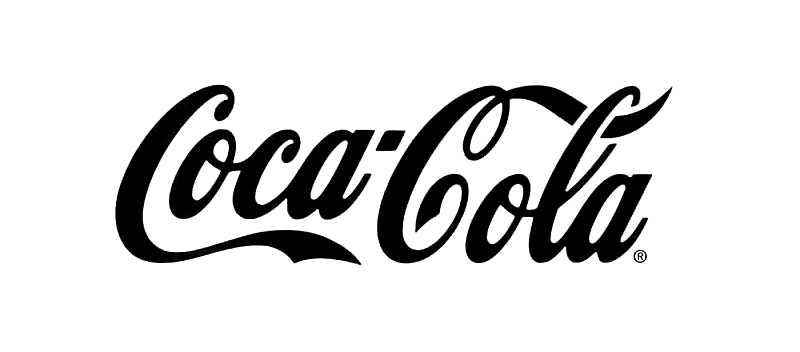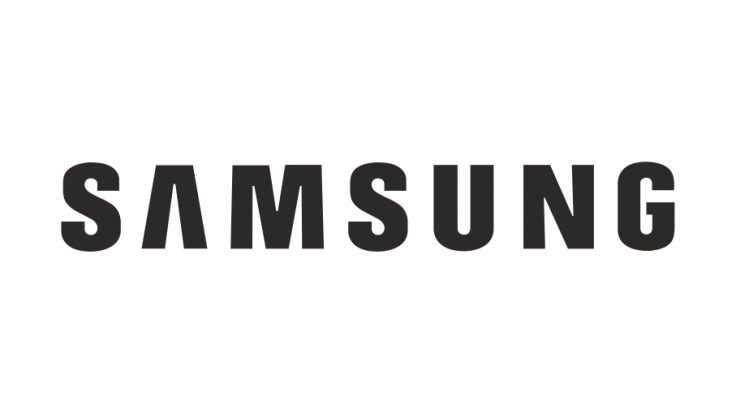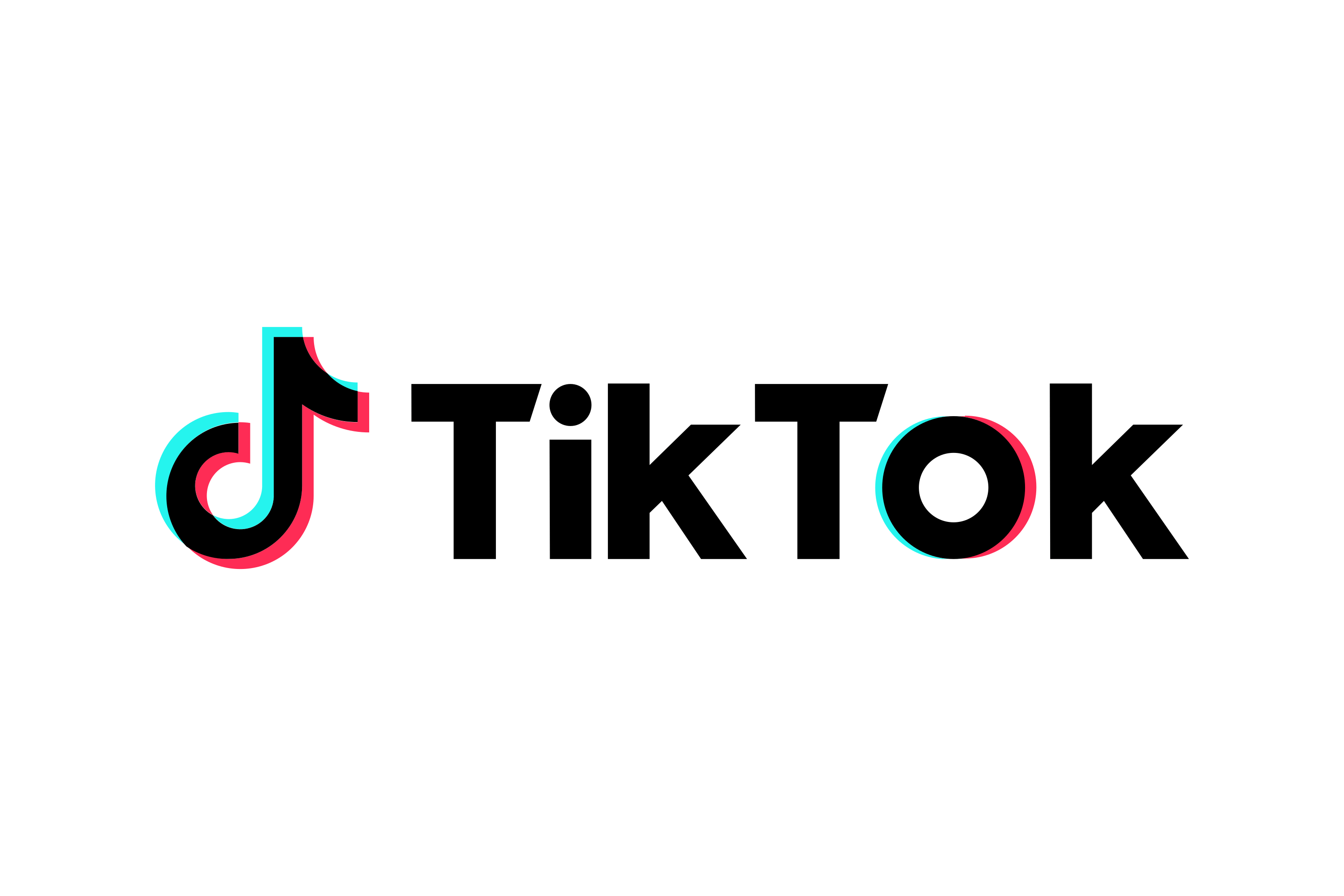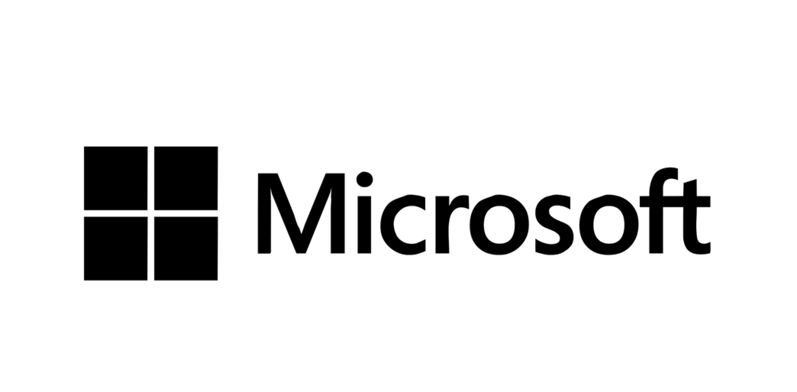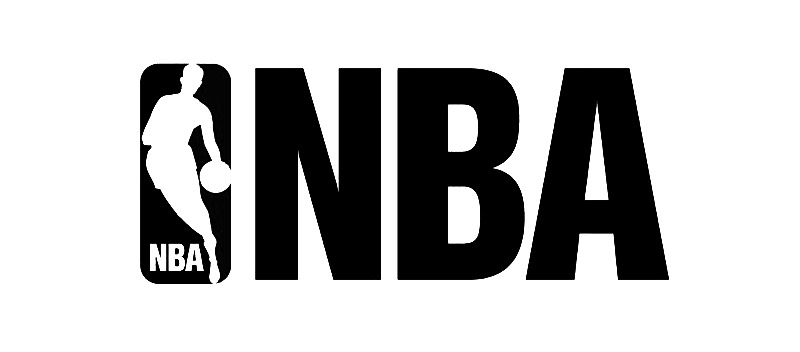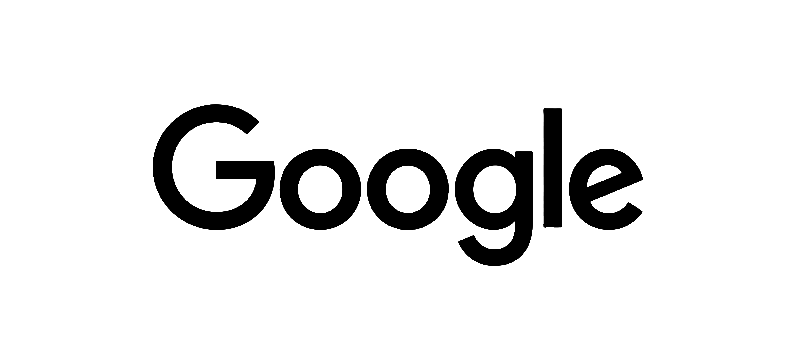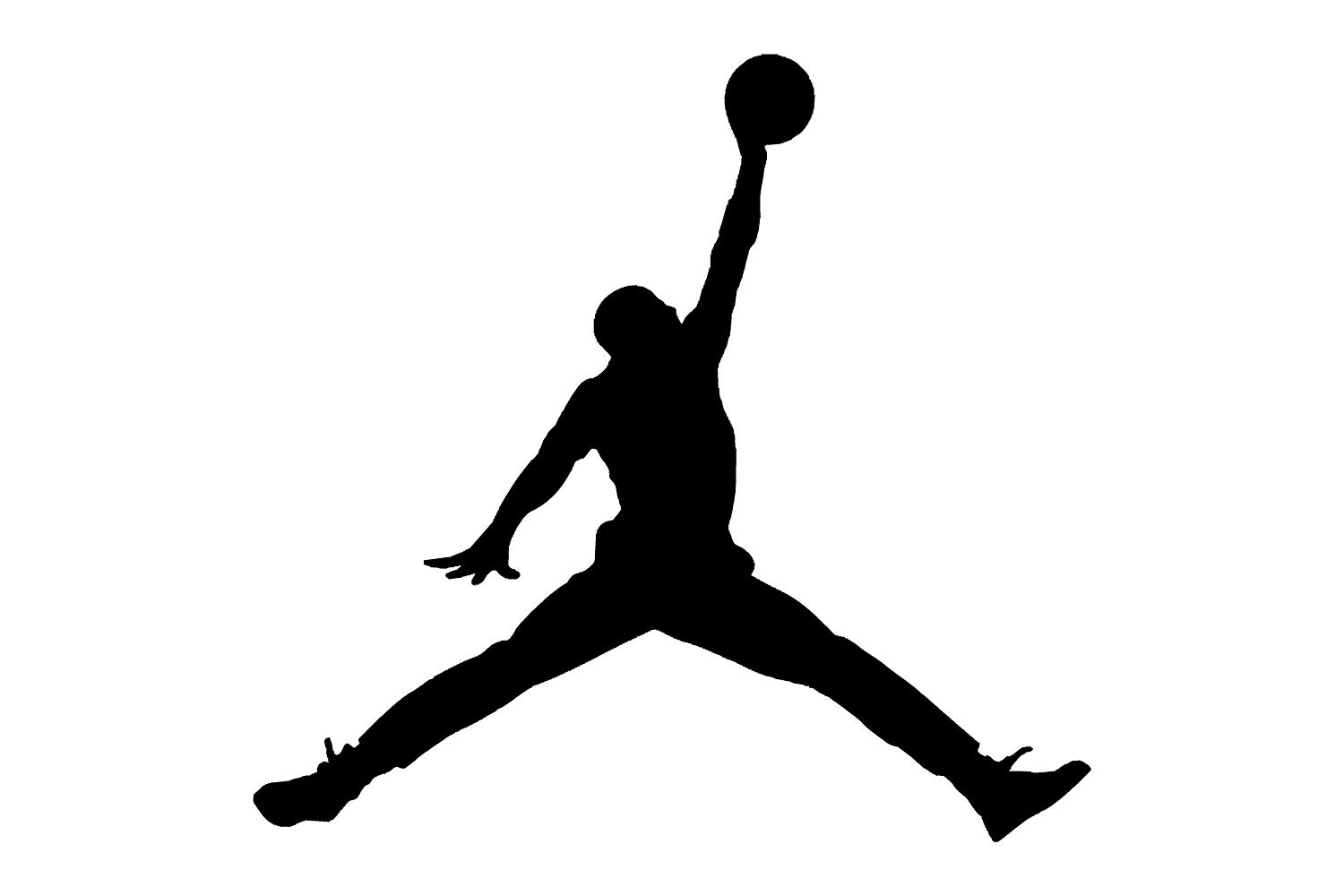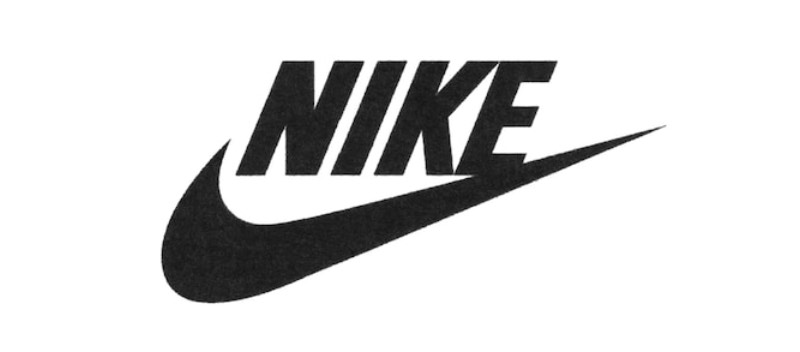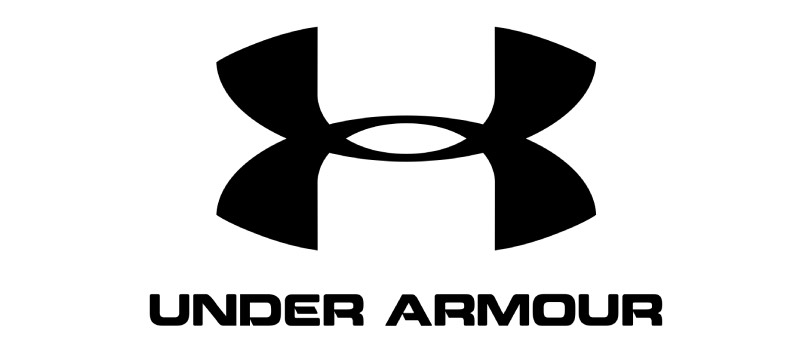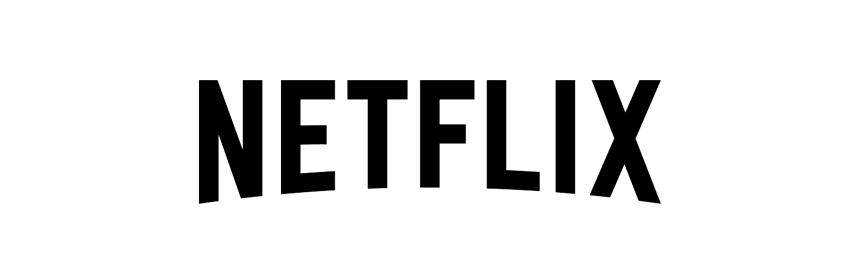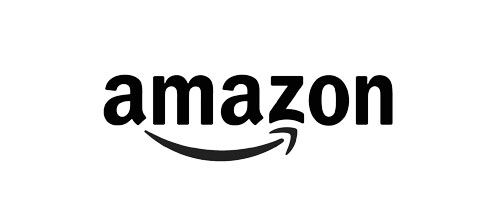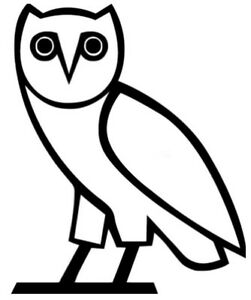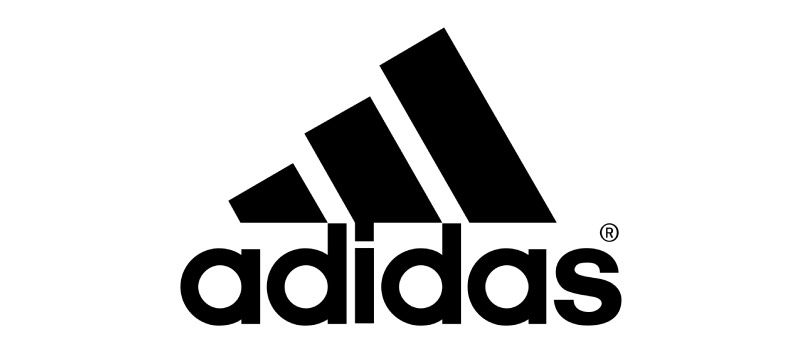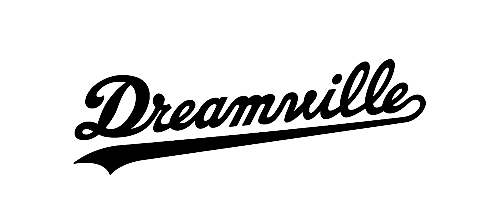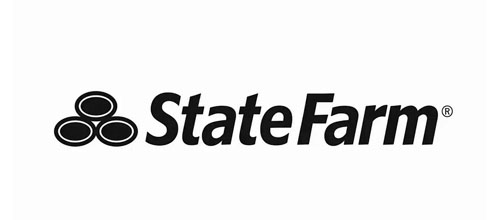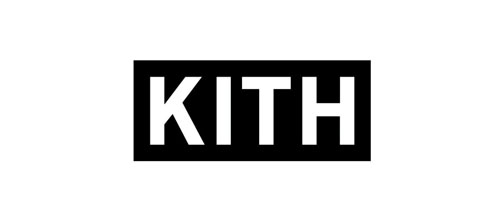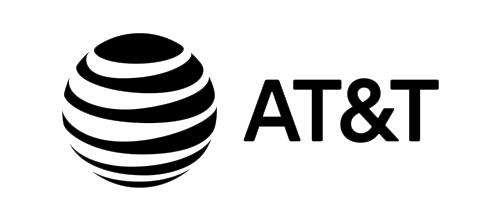LET'S WORK TOGETHER. LET'S WORK TOGETHER. LET'S WORK TOGETHER. LET'S WORK TOGETHER. LET'S WORK TOGETHER. LET'S WORK TOGETHER. LET'S WORK TOGETHER. LET'S WORK TOGETHER. LET'S WORK TOGETHER. LET'S WORK TOGETHER. LET'S WORK TOGETHER. LET'S WORK TOGETHER. LET'S WORK TOGETHER. LET'S WORK TOGETHER. LET'S WORK TOGETHER. LET'S WORK TOGETHER. LET'S WORK TOGETHER. LET'S WORK TOGETHER. LET'S WORK TOGETHER. LET'S WORK TOGETHER. LET'S WORK TOGETHER. LET'S WORK TOGETHER. LET'S WORK TOGETHER. LET'S WORK TOGETHER. LET'S WORK TOGETHER. LET'S WORK TOGETHER. LET'S WORK TOGETHER. LET'S WORK TOGETHER. LET'S WORK TOGETHER. LET'S WORK TOGETHER. LET'S WORK TOGETHER. LET'S WORK TOGETHER. LET'S WORK TOGETHER. LET'S WORK TOGETHER. LET'S WORK TOGETHER. LET'S WORK TOGETHER. LET'S WORK TOGETHER. LET'S WORK TOGETHER. LET'S WORK TOGETHER. LET'S WORK TOGETHER. LET'S WORK TOGETHER. LET'S WORK TOGETHER. LET'S WORK TOGETHER. LET'S WORK TOGETHER. LET'S WORK TOGETHER. LET'S WORK TOGETHER. LET'S WORK TOGETHER. LET'S WORK TOGETHER. LET'S WORK TOGETHER. LET'S WORK TOGETHER. LET'S WORK TOGETHER. LET'S WORK TOGETHER. LET'S WORK TOGETHER. LET'S WORK TOGETHER. LET'S WORK TOGETHER. LET'S WORK TOGETHER. LET'S WORK TOGETHER. LET'S WORK TOGETHER. LET'S WORK TOGETHER. LET'S WORK TOGETHER. LET'S WORK TOGETHER. LET'S WORK TOGETHER. LET'S WORK TOGETHER. LET'S WORK TOGETHER. LET'S WORK TOGETHER. LET'S WORK TOGETHER. LET'S WORK TOGETHER. LET'S WORK TOGETHER. LET'S WORK TOGETHER. LET'S WORK TOGETHER. LET'S WORK TOGETHER. LET'S WORK TOGETHER. LET'S WORK TOGETHER. LET'S WORK TOGETHER. LET'S WORK TOGETHER. LET'S WORK TOGETHER. LET'S WORK TOGETHER. LET'S WORK TOGETHER. LET'S WORK TOGETHER. LET'S WORK TOGETHER. LET'S WORK TOGETHER. LET'S WORK TOGETHER. LET'S WORK TOGETHER. LET'S WORK TOGETHER. LET'S WORK TOGETHER. LET'S WORK TOGETHER. LET'S WORK TOGETHER. LET'S WORK TOGETHER. LET'S WORK TOGETHER. LET'S WORK TOGETHER. LET'S WORK TOGETHER. LET'S WORK TOGETHER. LET'S WORK TOGETHER. LET'S WORK TOGETHER. LET'S WORK TOGETHER. LET'S WORK TOGETHER. LET'S WORK TOGETHER. LET'S WORK TOGETHER. LET'S WORK TOGETHER. LET'S WORK TOGETHER. LET'S WORK TOGETHER. LET'S WORK TOGETHER. LET'S WORK TOGETHER. LET'S WORK TOGETHER. LET'S WORK TOGETHER. LET'S WORK TOGETHER. LET'S WORK TOGETHER. LET'S WORK TOGETHER. LET'S WORK TOGETHER. LET'S WORK TOGETHER. LET'S WORK TOGETHER. LET'S WORK TOGETHER. LET'S WORK TOGETHER. LET'S WORK TOGETHER. LET'S WORK TOGETHER. LET'S WORK TOGETHER. LET'S WORK TOGETHER. LET'S WORK TOGETHER. LET'S WORK TOGETHER. LET'S WORK TOGETHER. LET'S WORK TOGETHER. LET'S WORK TOGETHER. LET'S WORK TOGETHER. LET'S WORK TOGETHER. LET'S WORK TOGETHER. LET'S WORK TOGETHER. LET'S WORK TOGETHER. LET'S WORK TOGETHER. LET'S WORK TOGETHER. LET'S WORK TOGETHER. LET'S WORK TOGETHER. LET'S WORK TOGETHER. LET'S WORK TOGETHER. LET'S WORK TOGETHER. LET'S WORK TOGETHER. LET'S WORK TOGETHER. LET'S WORK TOGETHER. LET'S WORK TOGETHER. LET'S WORK TOGETHER. LET'S WORK TOGETHER. LET'S WORK TOGETHER. LET'S WORK TOGETHER. LET'S WORK TOGETHER. LET'S WORK TOGETHER. LET'S WORK TOGETHER. LET'S WORK TOGETHER. LET'S WORK TOGETHER. LET'S WORK TOGETHER. LET'S WORK TOGETHER. LET'S WORK TOGETHER. LET'S WORK TOGETHER. LET'S WORK TOGETHER. LET'S WORK TOGETHER. LET'S WORK TOGETHER. LET'S WORK TOGETHER. LET'S WORK TOGETHER. LET'S WORK TOGETHER. LET'S WORK TOGETHER. LET'S WORK TOGETHER. LET'S WORK TOGETHER. LET'S WORK TOGETHER. LET'S WORK TOGETHER. LET'S WORK TOGETHER. LET'S WORK TOGETHER. LET'S WORK TOGETHER. LET'S WORK TOGETHER. LET'S WORK TOGETHER. LET'S WORK TOGETHER. LET'S WORK TOGETHER. LET'S WORK TOGETHER. LET'S WORK TOGETHER. LET'S WORK TOGETHER. LET'S WORK TOGETHER. LET'S WORK TOGETHER. LET'S WORK TOGETHER. LET'S WORK TOGETHER. LET'S WORK TOGETHER. LET'S WORK TOGETHER. LET'S WORK TOGETHER. LET'S WORK TOGETHER. LET'S WORK TOGETHER. LET'S WORK TOGETHER. LET'S WORK TOGETHER. LET'S WORK TOGETHER. LET'S WORK TOGETHER. LET'S WORK TOGETHER. LET'S WORK TOGETHER. LET'S WORK TOGETHER. LET'S WORK TOGETHER. LET'S WORK TOGETHER. LET'S WORK TOGETHER. LET'S WORK TOGETHER. LET'S WORK TOGETHER. LET'S WORK TOGETHER. LET'S WORK TOGETHER. LET'S WORK TOGETHER. LET'S WORK TOGETHER. LET'S WORK TOGETHER. LET'S WORK TOGETHER. LET'S WORK TOGETHER. LET'S WORK TOGETHER. LET'S WORK TOGETHER. LET'S WORK TOGETHER. LET'S WORK TOGETHER. LET'S WORK TOGETHER. LET'S WORK TOGETHER. LET'S WORK TOGETHER. LET'S WORK TOGETHER. LET'S WORK TOGETHER. LET'S WORK TOGETHER. LET'S WORK TOGETHER. LET'S WORK TOGETHER. LET'S WORK TOGETHER. LET'S WORK TOGETHER. LET'S WORK TOGETHER. LET'S WORK TOGETHER. LET'S WORK TOGETHER. LET'S WORK TOGETHER. LET'S WORK TOGETHER.LET'S WORK TOGETHER. LET'S WORK TOGETHER. LET'S WORK TOGETHER. LET'S WORK TOGETHER. LET'S WORK TOGETHER. LET'S WORK TOGETHER. LET'S WORK TOGETHER. LET'S WORK TOGETHER. LET'S WORK TOGETHER. LET'S WORK TOGETHER. LET'S WORK TOGETHER. LET'S WORK TOGETHER. LET'S WORK TOGETHER. LET'S WORK TOGETHER. LET'S WORK TOGETHER. LET'S WORK TOGETHER. LET'S WORK TOGETHER. LET'S WORK TOGETHER. LET'S WORK TOGETHER. LET'S WORK TOGETHER. LET'S WORK TOGETHER. LET'S WORK TOGETHER. LET'S WORK TOGETHER. LET'S WORK TOGETHER. LET'S WORK TOGETHER. LET'S WORK TOGETHER. LET'S WORK TOGETHER. LET'S WORK TOGETHER. LET'S WORK TOGETHER. LET'S WORK TOGETHER. LET'S WORK TOGETHER. LET'S WORK TOGETHER. SAY HELLO CONTACT@ISHAANMISHRA.COM ✉️ SAY HELLO CONTACT@ISHAANMISHRA.COM ✉️ SAY HELLO CONTACT@ISHAANMISHRA.COM ✉️ SAY HELLO CONTACT@ISHAANMISHRA.COM ✉️ SAY HELLO CONTACT@ISHAANMISHRA.COM ✉️ SAY HELLO CONTACT@ISHAANMISHRA.COM ✉️ SAY HELLO CONTACT@ISHAANMISHRA.COM ✉️ SAY HELLO CONTACT@ISHAANMISHRA.COM ✉️ SAY HELLO CONTACT@ISHAANMISHRA.COM ✉️ SAY HELLO CONTACT@ISHAANMISHRA.COM ✉️ SAY HELLO CONTACT@ISHAANMISHRA.COM ✉️ SAY HELLO CONTACT@ISHAANMISHRA.COM ✉️ SAY HELLO CONTACT@ISHAANMISHRA.COM ✉️ SAY HELLO CONTACT@ISHAANMISHRA.COM ✉️ SAY HELLO CONTACT@ISHAANMISHRA.COM ✉️ SAY HELLO CONTACT@ISHAANMISHRA.COM ✉️ SAY HELLO CONTACT@ISHAANMISHRA.COM ✉️ SAY HELLO CONTACT@ISHAANMISHRA.COM ✉️ SAY HELLO CONTACT@ISHAANMISHRA.COM ✉️ SAY HELLO CONTACT@ISHAANMISHRA.COM ✉️ SAY HELLO CONTACT@ISHAANMISHRA.COM ✉️ SAY HELLO CONTACT@ISHAANMISHRA.COM ✉️ SAY HELLO CONTACT@ISHAANMISHRA.COM ✉️ SAY HELLO CONTACT@ISHAANMISHRA.COM ✉️ SAY HELLO CONTACT@ISHAANMISHRA.COM ✉️ SAY HELLO CONTACT@ISHAANMISHRA.COM ✉️ SAY HELLO CONTACT@ISHAANMISHRA.COM ✉️ SAY HELLO CONTACT@ISHAANMISHRA.COM ✉️ SAY HELLO CONTACT@ISHAANMISHRA.COM ✉️ SAY HELLO CONTACT@ISHAANMISHRA.COM ✉️ SAY HELLO CONTACT@ISHAANMISHRA.COM ✉️ SAY HELLO CONTACT@ISHAANMISHRA.COM ✉️ SAY HELLO CONTACT@ISHAANMISHRA.COM ✉️ SAY HELLO CONTACT@ISHAANMISHRA.COM ✉️ SAY HELLO CONTACT@ISHAANMISHRA.COM ✉️ SAY HELLO CONTACT@ISHAANMISHRA.COM ✉️ SAY HELLO CONTACT@ISHAANMISHRA.COM ✉️ SAY HELLO CONTACT@ISHAANMISHRA.COM ✉️ SAY HELLO CONTACT@ISHAANMISHRA.COM ✉️ SAY HELLO CONTACT@ISHAANMISHRA.COM ✉️ SAY HELLO CONTACT@ISHAANMISHRA.COM ✉️ SAY HELLO CONTACT@ISHAANMISHRA.COM ✉️ SAY HELLO CONTACT@ISHAANMISHRA.COM ✉️ SAY HELLO CONTACT@ISHAANMISHRA.COM ✉️ SAY HELLO CONTACT@ISHAANMISHRA.COM ✉️ SAY HELLO CONTACT@ISHAANMISHRA.COM ✉️ SAY HELLO CONTACT@ISHAANMISHRA.COM ✉️ SAY HELLO CONTACT@ISHAANMISHRA.COM ✉️ SAY HELLO CONTACT@ISHAANMISHRA.COM ✉️ SAY HELLO CONTACT@ISHAANMISHRA.COM ✉️ SAY HELLO CONTACT@ISHAANMISHRA.COM ✉️ SAY HELLO CONTACT@ISHAANMISHRA.COM ✉️ SAY HELLO CONTACT@ISHAANMISHRA.COM ✉️ SAY HELLO CONTACT@ISHAANMISHRA.COM ✉️ SAY HELLO CONTACT@ISHAANMISHRA.COM ✉️ SAY HELLO CONTACT@ISHAANMISHRA.COM ✉️ SAY HELLO CONTACT@ISHAANMISHRA.COM ✉️ SAY HELLO CONTACT@ISHAANMISHRA.COM ✉️ LET'S WORK TOGETHER. LET'S WORK TOGETHER. LET'S WORK TOGETHER. LET'S WORK TOGETHER. LET'S WORK TOGETHER. LET'S WORK TOGETHER. LET'S WORK TOGETHER. LET'S WORK TOGETHER. LET'S WORK TOGETHER. LET'S WORK TOGETHER. LET'S WORK TOGETHER. LET'S WORK TOGETHER. LET'S WORK TOGETHER. LET'S WORK TOGETHER. LET'S WORK TOGETHER. LET'S WORK TOGETHER. LET'S WORK TOGETHER. LET'S WORK TOGETHER. LET'S WORK TOGETHER. LET'S WORK TOGETHER. LET'S WORK TOGETHER. LET'S WORK TOGETHER. LET'S WORK TOGETHER. LET'S WORK TOGETHER. LET'S WORK TOGETHER. LET'S WORK TOGETHER. LET'S WORK TOGETHER. LET'S WORK TOGETHER. LET'S WORK TOGETHER. LET'S WORK TOGETHER. LET'S WORK TOGETHER. LET'S WORK TOGETHER. LET'S WORK TOGETHER. LET'S WORK TOGETHER. LET'S WORK TOGETHER. LET'S WORK TOGETHER. LET'S WORK TOGETHER. LET'S WORK TOGETHER. LET'S WORK TOGETHER. LET'S WORK TOGETHER. LET'S WORK TOGETHER. LET'S WORK TOGETHER. LET'S WORK TOGETHER. LET'S WORK TOGETHER. LET'S WORK TOGETHER. LET'S WORK TOGETHER. LET'S WORK TOGETHER. LET'S WORK TOGETHER. LET'S WORK TOGETHER. LET'S WORK TOGETHER. LET'S WORK TOGETHER. LET'S WORK TOGETHER. LET'S WORK TOGETHER. LET'S WORK TOGETHER. LET'S WORK TOGETHER. LET'S WORK TOGETHER. LET'S WORK TOGETHER. LET'S WORK TOGETHER. LET'S WORK TOGETHER. LET'S WORK TOGETHER. LET'S WORK TOGETHER. LET'S WORK TOGETHER. LET'S WORK TOGETHER. LET'S WORK TOGETHER. LET'S WORK TOGETHER. LET'S WORK TOGETHER. LET'S WORK TOGETHER. LET'S WORK TOGETHER. LET'S WORK TOGETHER. LET'S WORK TOGETHER. LET'S WORK TOGETHER. LET'S WORK TOGETHER. LET'S WORK TOGETHER. LET'S WORK TOGETHER. LET'S WORK TOGETHER. LET'S WORK TOGETHER. LET'S WORK TOGETHER. LET'S WORK TOGETHER. LET'S WORK TOGETHER. LET'S WORK TOGETHER. LET'S WORK TOGETHER. LET'S WORK TOGETHER. LET'S WORK TOGETHER. LET'S WORK TOGETHER. LET'S WORK TOGETHER. LET'S WORK TOGETHER. LET'S WORK TOGETHER. LET'S WORK TOGETHER. LET'S WORK TOGETHER. LET'S WORK TOGETHER. LET'S WORK TOGETHER. LET'S WORK TOGETHER. LET'S WORK TOGETHER. LET'S WORK TOGETHER. LET'S WORK TOGETHER. LET'S WORK TOGETHER. LET'S WORK TOGETHER. LET'S WORK TOGETHER.

## CONTACT

### SAY HELLO.DROP MEA LINE.

Oops! Something went wrong while submitting the form.

## FAQs 🤔

How do you approach design projects?

When I embark on a design project, I enter into a realm of infinite possibility and boundless creativity. At the outset, I seek to immerse myself in the unique context of the project, whether it be the client's brand identity, the market landscape, or the cultural zeitgeist. Through this deep immersion, I am able to distill the essence of the challenge at hand, uncover the hidden nuances, and unearth the unspoken desires.

How do you determine the audience to design for?

As I refine and iterate on my ideas, I strive to maintain a laser focus on the user experience, putting myself in the shoes of the end user and designing with their needs and desires at the forefront of my mind. I believe that design is not just about creating something beautiful or functional, but about enhancing and enriching the human experience.

What sets you apart from other creative directors?

One key differentiator is my ability to seamlessly integrate form and function in my designs. I believe that true innovation lies at the intersection of beauty and utility, and I strive to create designs that not only look stunning but also enhance and enrich the user experience.

Another aspect that sets me apart is my emphasis on empathy and human connection. While many designers focus solely on aesthetics or functionality, I believe that the most successful designs are those that are deeply rooted in the needs and desires of the user. I see design not as an end in itself, but as a means of facilitating meaningful human interactions and experiences.

Ultimately, differentiating my creative voice within the landscape is about being curious, open-minded, and committed to lifelong learning. By remaining engaged and curious, I am able to continuously push the boundaries of what is possible in design and deliver innovative, impactful solutions for my partners and clients.

How do you stay up-to-date with design trends?

To stay current and informed, I engage in a number of activities that allow me to learn, explore, and experiment with new ideas and approaches. I make it a priority to stay connected to the broader design community. I stay current on the latest software and hardware releases, and I invest time in exploring emerging technologies such as virtual and augmented reality.

I also stay informed about new materials and manufacturing techniques that can impact the design process.I also believe that it's important to draw inspiration from outside the world of design. I engage with other fields, such as art, science, and technology, to gain new perspectives and insights that can inform my design work. I also make a point to explore different cultures and travel to new places, which can provide fresh inspiration and new perspectives on design.

What types of projects do you specialize in?

I specialize in a wide range of design projects that span multiple disciplines and platforms. Some of the areas that I excel in include:

Product Design: I have a deep expertise in designing physical products, from consumer electronics to furniture to fashion. I am skilled in crafting products that are not only beautiful but also functional and user-friendly.

Digital Design: I have extensive experience in designing digital experiences, including websites, mobile apps, and other digital platforms. I am skilled in UX/UI design, information architecture, and user testing.

AR/VR Design: I have a passion for exploring emerging technologies such as augmented and virtual reality, and I have experience in designing immersive experiences that leverage these technologies.

Video Editing: I have a strong background in video editing and production, and I am skilled in creating compelling visual narratives that tell a story and connect with audiences.

Creative Direction and Art Direction: I have a talent for bringing together diverse creative teams and providing guidance and direction to ensure a cohesive vision and execution. I am experienced in art direction, photography, and graphic design.

Social Media: I am adept at creating engaging content and campaigns for social media platforms, and I understand how to leverage social media to build brand awareness and drive engagement.

What design software and tools do you use?

I have extensive experience working with a wide range of design software and tools. I believe that each project requires a unique set of tools and techniques, so I am always exploring new software and technologies to find the best solutions for my clients.

Some of the tools that I use regularly include Adobe Creative Suite for graphic design, photo editing, and page layout, Sketch and Figma for collaborative user interface and user experience (UI/UX) design, Cinema 4D for 3D modeling and animation, After Effects and Cavalry for motion graphics, Procreate for digital sketching and drawing, and Final Cut Pro and Premiere Pro for video editing and production.

I also use Trello and Asana for project management and organization. I am always seeking out new tools and technologies that can enhance creativity, productivity, and collaboration, and I believe that staying current with the latest tools and techniques is essential to delivering innovative and impactful design solutions for my clients.